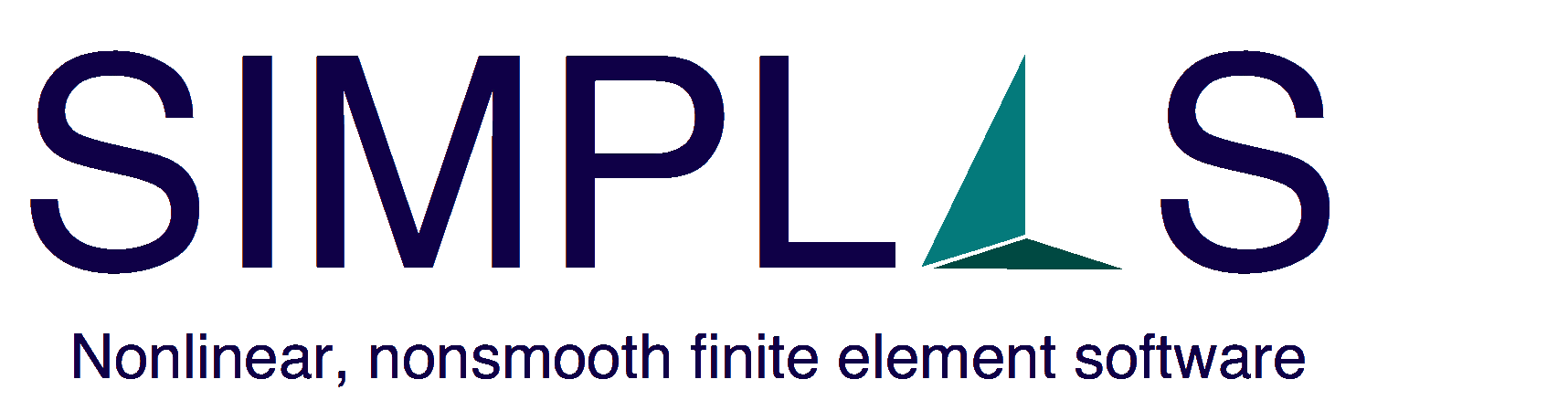# End-user features of SIMPLAS

In terms of features, we focus on intense testing and rigorous peer-review scrutiny instead of “state of the art”. In addition, we are concerned with orthogonality of features: all elements are compatible with all constitutive models. We also do not cut corners: calculations are performed as exactly as possible with certain elements possessing tens of thousands of lines of source code. Time integration with inertia is energy conserving and consistent mass matrices are used in all elements.
1. Solution choices
1. Linearized solution (strictly not linear).
2. Monotonous non-linear solution (quasi-static).
3. Monotonous non-linear solution (dynamic).
4. Linear control (quasi-static and dynamic).
5. Quadratic control (quasi-static and dynamic).
2. Solution stabilization and non-smooth treatment
1. Increment control (based on constitutive evolution and iteration history).
2. Step size control.
3. Chen-Mangasarian replacement function for non-smooth problems.
3. Constitutive models
1. Incremental Kirchhoff/Saint-Venant (isotropic and anisotropic).
2. Neo-Hookean (polyconvex version).
3. Total Kirchhoff/Saint-Venant.
4. Ogden.
5. Brittle damage model.
6. Elasto-plastic with von-Mises yield function.
7. Elasto-plastic with Hill anisotropic criterion.
8. Elasto-plastic GTN (Gurson Tvergaard Needleman).
9. Elasto-plastic Rousselier.
4. Finite strain discretization (elements)
1. Plane strain quadrilaterals (B-Bar and finite strain version of Pian-Sumihara).
2. Plane stress quadrilaterals (with thickness extensibility).
3. Axisymmetric quadrilaterals (B-Bar with mean circumferential strain)
4. Plane strain triangles (MINI).
5. Plane stress triangles (displacement-based).
6. Shell triangles (discrete Kirchhoff with drilling, corotational with exact polar decomposition).
7. Shell quadrilateral (assumed Natural Strain for transverse terms and Pian-Sumihara for in-plane terms).
8. Tetrahedra (displacement-based and MINI).
9. Hexahedra (B-Bar and Enhanced Assumed Strain).
5. Discretization (constraints)
1. Initial conditions.
2. Essential boundary conditions.
3. Equal degrees-of-freedom.
4. Rigid body constraints.